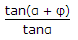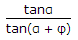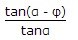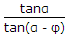# Mechanical Engineering - Engineering Mechanics

### Exercise :: Engineering Mechanics - Section 4

1.

Three forces acting on a rigid body are represented in magnitude, direction and line of action by the three sides of a triangle taken in order. The forces are equivalent to a couple whose moment is equal to

 A. area of the triangle B. twice the area of the triangle C. half the area of the triangle D. none of these

Explanation:

No answer description available for this question. Let us discuss.

2.

If tension in the cable supporting a lift moving downwards is half the tension when it is moving upwards, the acceleration of the lift is

 A. g/2 B. g/3 C. g/4 D. none of these

Explanation:

No answer description available for this question. Let us discuss.

3.

Efficiency of a screw jack is given by(where α = Helix angle, and φ = Angle of friction.)

 A.B.C.D.Explanation:

No answer description available for this question. Let us discuss.

4.

When a train is rounding a curve, the side thrust on the wheel flanges is prevented by raising the outer edge of the rail.

 A. Yes B. No

Explanation:

No answer description available for this question. Let us discuss.

5.

Frequency of vibrations means the number of cycles per second.

 A. Yes B. No